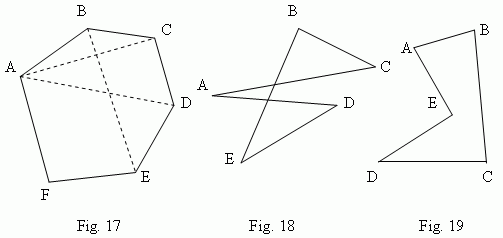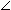# Polygon

Polygon. Vertices, angles, diagonals, sides of a polygon.
Perimeter of a polygon. Simple polygons. Convex polygon.
Sum of interior angles in a convex polygon.

A plane figure, formed by closed chain of segments, is called a polygon . Depending on a quantity of angles a polygon can be a triangle , a quadrangle , a pentagon , a hexagon etc. On  Fig.17  the hexagon ABCDEF is shown. PointsA, B, C, D, E, F – vertices of polygon ; anglesA ,B ,C ,D,E ,F – angles of polygon ; segments AC, AD, BE etc. are diagonals ; AB, BC, CD, DE, EF, FA – sides of polygon ; a sum of sides lengths AB + BC + … + FA is called a perimeter of polygon and signed as p (sometimes – 2 p , then p – a half-perimeter). We consider only simple polygons in an elementary geometry, contours of which have no self-intersections  ( as shown on Fig.18 ). If all diagonals lie inside of a polygon, it is called a convex polygon. A hexagon on  Fig.17 is a convex one;  a pentagon ABCDE on  Fig.19 is not a convex polygon, because its diagonal AD lies outside of it. A sum of interior angles in any convex polygon is equal to 180 ( n – 2 ) deg, where n is a number of angles (or sides) of a polygon.